Home Normal Version IE Version Search | BBS | Guest Book | Site Map | Help | Contact us | About us
 Light version

[Index]

Introduction
Essence
Memorizing
Understanding
Summarizing
Examples

Example 1
Example 2
Example 3
Example 4
Example 5
Example 6
Example 7
Example 8
General ideas

Exercises
Final thoughts
Page 1 | Page 2 | Page 3 | Page 4 | Proof(s)

### Example 3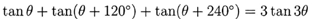We don't know if you already noticed that this is a hard one. Before we do anything else, let's find out what are the differences between the two sides. The angles and operations are different. Let's look at it closely. The right side is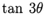. If you did read our Double & Triple Angle formulas in Memorizing the formulas section, you should know it equals to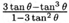. Believe it or not, you need to memorize it, or you will be in trouble, at least in this problem. Now the right side looks like this: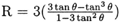We will not say we can't, but we think it's not a good idea to prove it from the right side to the left side.

Once we know the right side, we need to compare both sides again. Now, we see that they have two differences. It didn't change a lot, but, it's very easy to changeto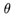and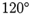, andis special angle, the tangent of which could be changed to a special number. So we use Sum formulas to do this.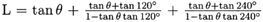Now, we want to change the values of functions of special angles to special numbers, so we leave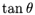there.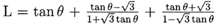We know that the right side is multiplication, so we want to change the operations of left side to multiplication too. If we simplify the left side, we get: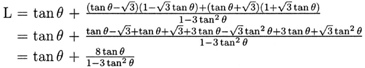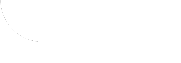LWR